# Assignment Problem

Use the solver in Excel to find the assignment of persons to tasks that minimizes the total cost.

### Formulate the Model

The model we are going to solve looks as follows in Excel.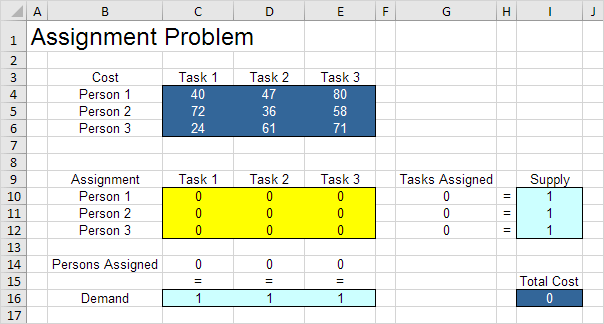1. To formulate this assignment problem, answer the following three questions.

a. What are the decisions to be made? For this problem, we need Excel to find out which person to assign to which task (Yes=1, No=0). For example, if we assign Person 1 to Task 1, cell C10 equals 1. If not, cell C10 equals 0.

b. What are the constraints on these decisions? Each person can only do one task (Supply=1). Each task only needs one person (Demand=1).

c. What is the overall measure of performance for these decisions? The overall measure of performance is the total cost of the assignment, so the objective is to minimize this quantity.

2. To make the model easier to understand, create the following named ranges.

Range Name Cells
Cost C4:E6
Assignment C10:E12
PersonsAssigned C14:E14
Demand C16:E16
Supply I10:I12
TotalCost I16

3. Insert the following functions.Explanation: The SUM functions calculate the number of tasks assigned to a person and the number of persons assigned to a task. Total Cost equals the sumproduct of Cost and Assignment.

### Trial and Error

With this formulation, it becomes easy to analyze any trial solution.

For example, if we assign Person 1 to Task 1, Person 2 to task 2 and Person 3 to Task 3, Tasks Assigned equals Supply and Persons Assigned equals Demand. This solution has a total cost of 147.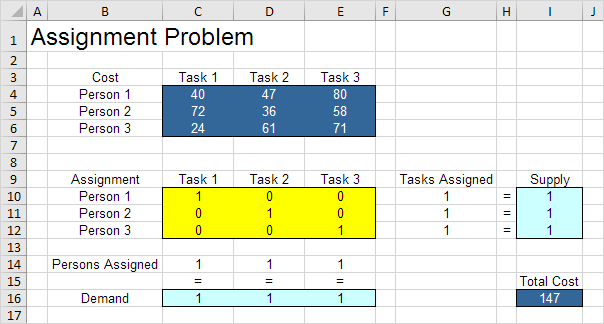It is not necessary to use trial and error. We shall describe next how the Excel Solver can be used to quickly find the optimal solution.

### Solve the Model

To find the optimal solution, execute the following steps.

1. On the Data tab, in the Analyze group, click Solver.Enter the solver parameters (read on). The result should be consistent with the picture below.You have the choice of typing the range names or clicking on the cells in the spreadsheet.

2. Enter TotalCost for the Objective.

3. Click Min.

4. Enter Assignment for the Changing Variable Cells.

5. Click Add to enter the following constraint.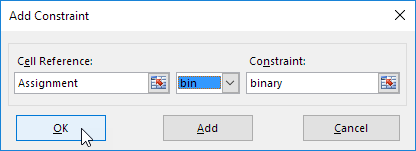Note: binary variables are either 0 or 1.

6. Click Add to enter the following constraint.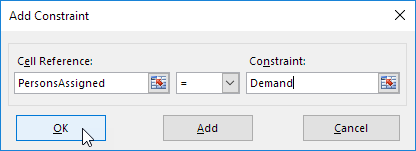7. Click Add to enter the following constraint.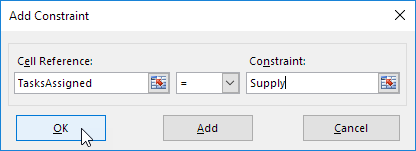8. Check 'Make Unconstrained Variables Non-Negative' and select 'Simplex LP'.

9. Finally, click Solve.

Result:The optimal solution:Conclusion: it is optimal to assign Person 1 to task 2, Person 2 to Task 3 and Person 3 to Task 1. This solution gives the minimum cost of 129. All constraints are satisfied.